Courses

# Test: Association & Dissociation

## 10 Questions MCQ Test Chemistry for JEE | Test: Association & Dissociation

Description
This mock test of Test: Association & Dissociation for JEE helps you for every JEE entrance exam. This contains 10 Multiple Choice Questions for JEE Test: Association & Dissociation (mcq) to study with solutions a complete question bank. The solved questions answers in this Test: Association & Dissociation quiz give you a good mix of easy questions and tough questions. JEE students definitely take this Test: Association & Dissociation exercise for a better result in the exam. You can find other Test: Association & Dissociation extra questions, long questions & short questions for JEE on EduRev as well by searching above.
QUESTION: 1

### The osmotic pressure of a 0.010 M MgSO4 solution at 500 K is 0.82 atm. Calculate , the van’t Hoff factor, for this MgSO4 solution.

Solution: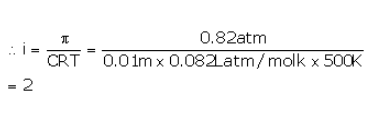QUESTION: 2

### Benzoic acid dissolved in benzene will show a molecular weight of

Solution:

In benzene, benzoic acid exist as dimer. So the molecular weight of benzoic acid gets doubled.

(C6​H5​COOH)2​=2×122=244 units .

QUESTION: 3

### Molar mass that is either lower or higher than expected or normal molar mass is called as

Solution:

The correct answer is option A
In chemistry, when the molar masses are calculated and if they are higher or lower than the expected value are known as abnormal molar masses. These are calculated using the colligative properties. Colligative properties include; elevation of boiling point, lowering of vapor pressure, freezing point depression, and ease of osmotic pressure. The name in itself has abnormal in it which suggests the abnormality of how the molar masses are being calculated, using the Van’t Hoff factor.

QUESTION: 4

0.01 M solution of common salt (NaCl) and (CH3COOH ) is taken. If their osmotic pressures are p1and p2 resp., what will be the correct statement correlating their osmotic pressures?

Solution:

The correct answer is Option B.

The osmotic pressure of the NaCl solution is more due to the Van't Hoff factor of NaCl.
Van't Hoff factor of NaCl is 2.
The Van't Hoff factor of acetic acid is between 1 and 2.
Hence P17P2

QUESTION: 5

If α is the degree of dissociation of Na2SO4, the vant Hoff’s factor (i) used for calculating the molecular mass is

Solution: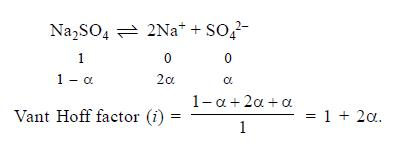QUESTION: 6

Lowering of vapour pressure is highest for

Solution: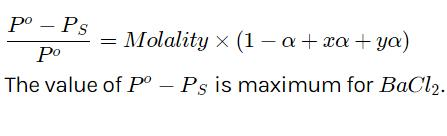QUESTION: 7

Increasing the temperature of solution of a weak electrolyte will cause

Solution:

The correct answer is option D

Increase in the temperature of solution of a weak electrolyte will result in an increase in degree of dissociation causing increase in
van’t Hoff factor.

QUESTION: 8

What is the freezing point of 0.1 m glucose solution in water? For water Kf is 1.86 K kg/mol?

Solution:

The correct answer is option C
Since glucose is a nonelectrolyte the van’t Hoff factor i = 1
ΔTf= iKfm
=1.86 x 0.1
=0.186
0oC – Tf =0.186
Tf= - 0.186oC

QUESTION: 9

van’t Hoff factor is denoted by letter

Solution:

The correct answer is option A
The Van't Hoff Factor is denoted by the letter 'i'. Where abnormal molar mass is determined experimentally and colligative properties are obtained by keeping in mind that solute (non-volatile) is neither associated or dissociated.
The Van’t Hoff Factor is denoted by the letter ‘i’.
Van’t Hoff defined this factor as follows: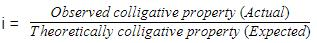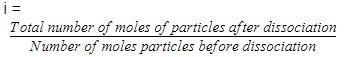Where abnormal molar mass is determined experimentally and colligative properties are obtained by keeping in mind that solute (non-volatile) is neither associated or dissociated.
In the case of dissociation i < 1.
And in the case of association i > .
Thus, equations for colligative properties can be modified as follows after inclusion of Van’t Hoff factors.
Relative lowering of vapour pressure of solvent
(p01 - p1)/p01  = i.(n2/n1)
Elevation of boiling point,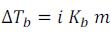Depression of Freezing point,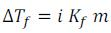Osmotic pressure of solutions,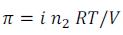QUESTION: 10

The ratio of osmotic pressure of a 1:1 electrolyte AB to that of a non electrolyte solute of same concentration is

Solution:

The correct answer is Option A.
The Van't Hoff factor of 1:1 electrolyte is 2 since it dissociates into two ions. van’t Hoff factor of a non electrolyte is 1 since it does not dissociate into ions. Hence ratio of osmotic pressure will be 2:1

• Test